# 01. Java development environment construction

## 0101. Java installation

### 010102. Please install according to the procedure manual.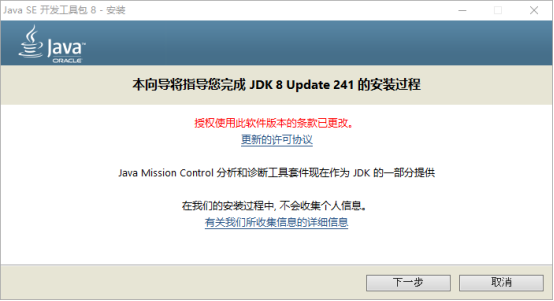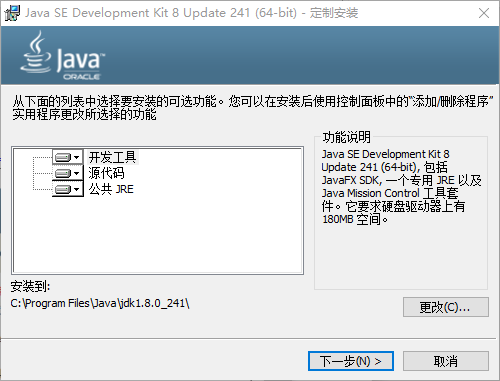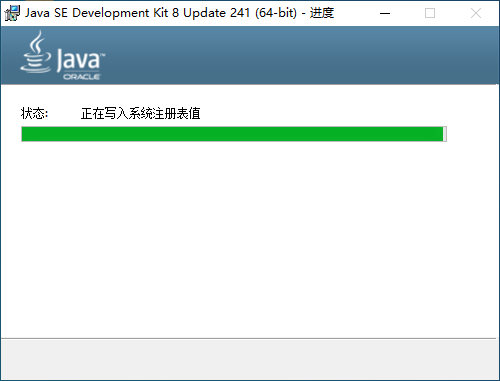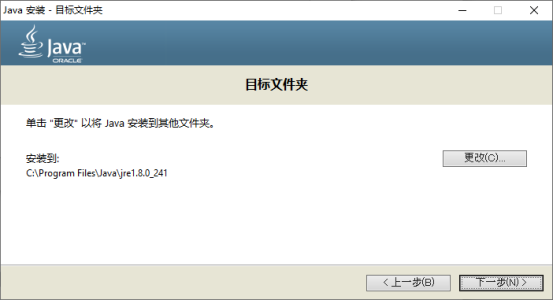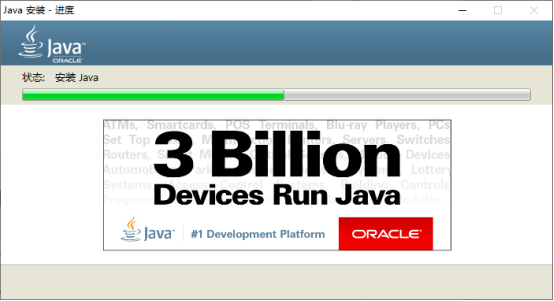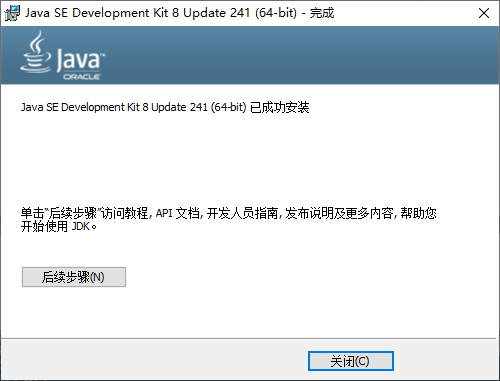### 010103. JAVA_HOME setting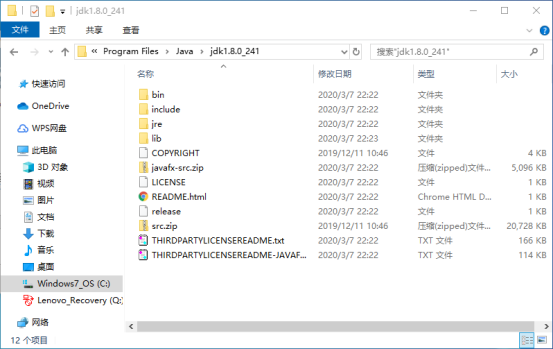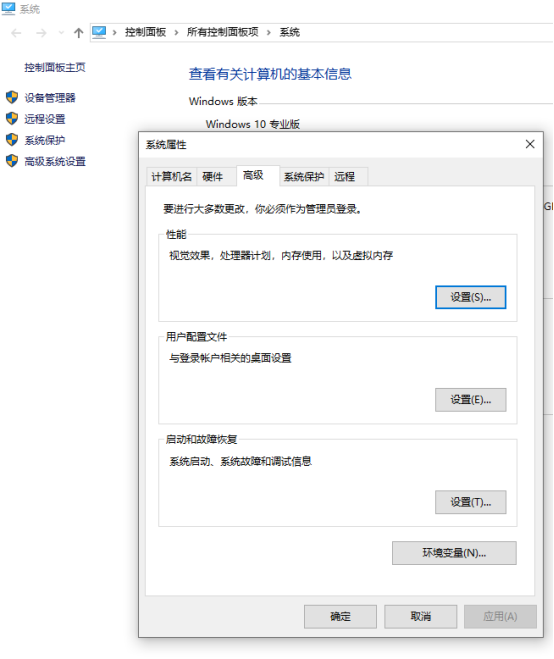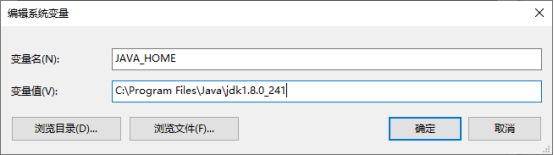## 0102. IDE installation

### 010202. Please install according to the procedure manual.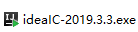のダブルクリック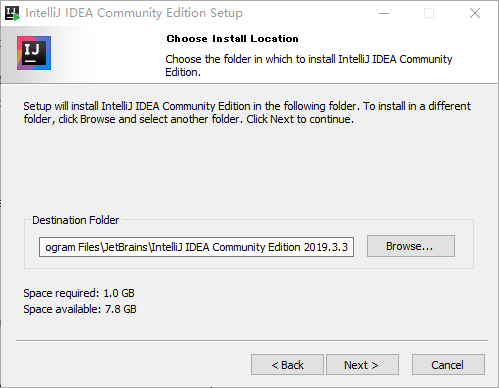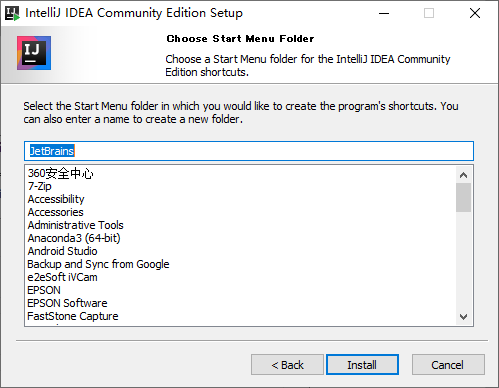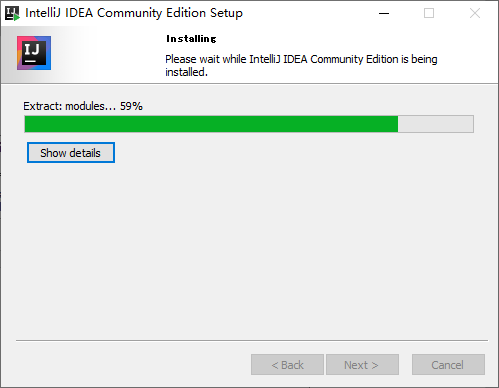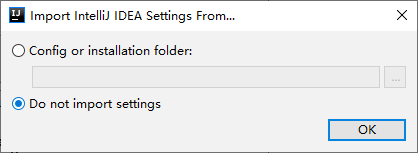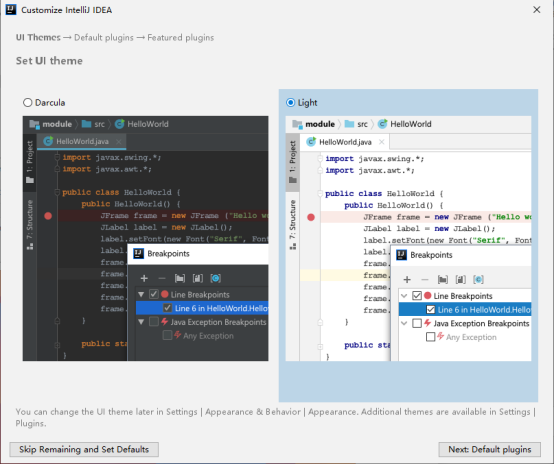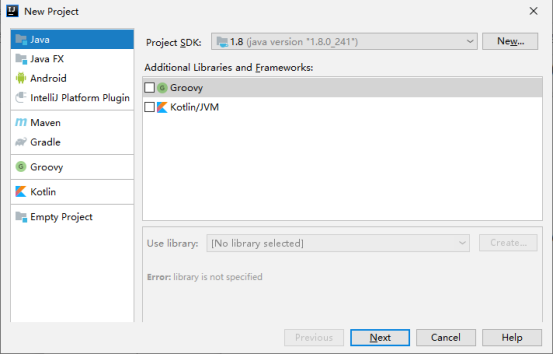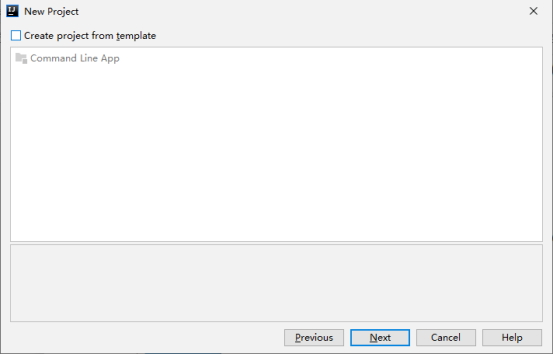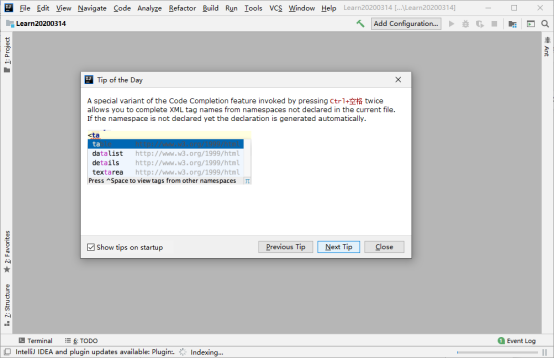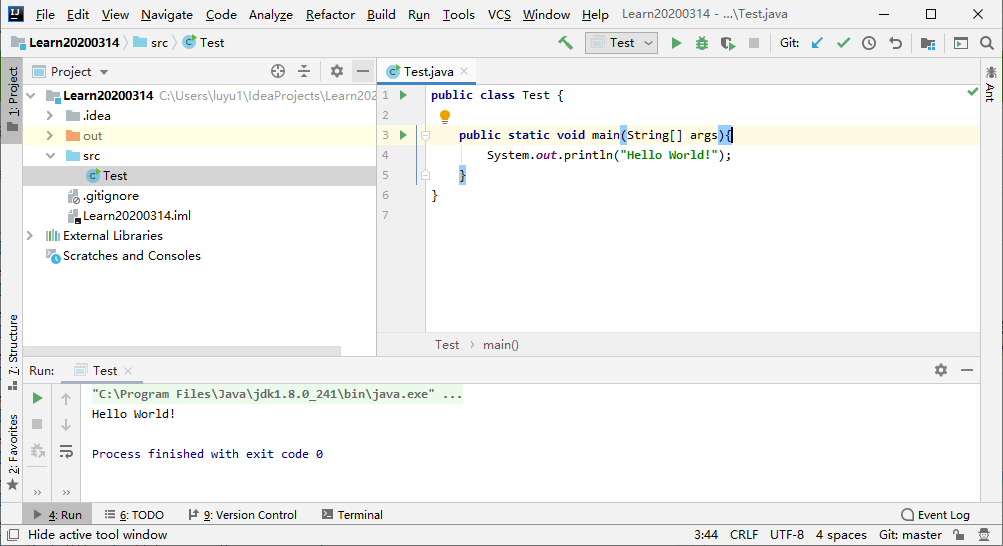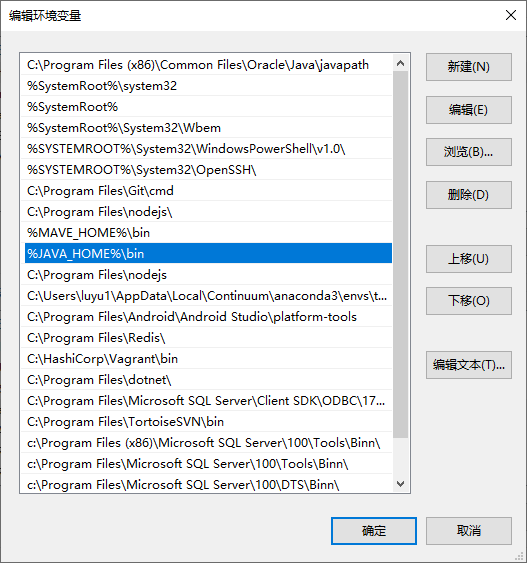### 010203. Other

--Eclipsen Japanese localization (https://mergedoc.osdn.jp/)

• VSCODE(https://code.visualstudio.com/docs/?dv=win64user)

# 02. Java basics

## 0201.Java-Basic syntax

■ When thinking about Java programs, there are the following elements.

• object --Class --Method --Instance variables

■ First Java program

#### `java`

``````
public class Test {
/**
* First program
* @param args
*/
public static void main(String []args){
System.out.println("Hello World!");
}
}
``````

#### `cmd`

``````
C:\Users\luyu1\IdeaProjects\Learn20200314\src>javac Test.java
C:\Users\luyu1\IdeaProjects\Learn20200314\src>java Test
``````

■ Basic syntax For Java programs, it is very important to keep the following in mind:

--Case sensitive --Class name (from uppercase) --Method name (from lowercase) --Class name = save file name --Execution entrance (public static void main (String args []))

■ Java identifier In Java, there are some points to keep in mind about identifiers.

--All identifiers must start with a letter (A to Z or a to z), a currency letter (\$), or an underscore (_).

--The identifier can have any combination of characters after the first character.

--Keywords cannot be used as identifiers.

――The most important thing is that identifiers are case sensitive.

--Examples of valid identifiers: age, \$ salary, _value, __ 1_value.

--Examples of incorrect identifiers: 123abc, -salary.

#### `java`

``````
``````

■ Java modifier There are two categories of modifiers.

--Accessible modifiers --default, public, protected, private

--Inaccessible modifiers − final, abstract, strictfp

#### `java`

``````
``````

■ Java variables Below are the Java variable types

--Local variables --Class variables (static variables) --Instance variables (non-static variables)

#### `java`

``````
``````

■ Java array An array is an object that stores multiple variables of the same type.

■ Java enumeration

#### `java`

``````
class FreshJuice {
enum FreshJuiceSize{ SMALL, MEDIUM, LARGE }
FreshJuiceSize size;
}

public class FreshJuiceTest {

public static void main(String args[]) {
FreshJuice juice = new FreshJuice();
juice.size = FreshJuice.FreshJuiceSize.MEDIUM ;
System.out.println("Size: " + juice.size);
}
}
``````

■ Java keywords The following list shows Java reserved words.

keyword keyword keyword keyword
abstract assert boolean break
byte case catch char
class const continue default
do double else enum
extends final finally float
for goto if implements
import instanceof int interface
long native new package
private protected public return
short static strictfp super
switch synchronized this throw
throws transient try void
volatile while

■ Java comments Supports single-line and multi-line comments. All characters available in the comment are ignored by the Java compiler.

#### `java`

``````
public class MyFirstJavaProgram {

/* This is my first java program.
* This will print 'Hello World' as the output
* This is an example of multi-line comments.
*/

public static void main(String []args) {
// This is an example of single line comment
/* This is also an example of single line comment. */
System.out.println("Hello World");
}
}
``````

■ Use blank lines Lines that probably contain only commented whitespace are called blank lines, and Java ignores them altogether.

■ Inheritance In Java, classes can be derived from classes. Basically, if you need to create a new class and it already contains some of the code you need, you can derive the new class from your existing code. In this scenario, existing classes are called superclasses and derived classes are called subclasses.

■ Interface In the Java language, interfaces can be defined as contracts about how objects communicate with each other. Interfaces play an important role in the concept of inheritance.

The interface defines methods and must be used by derived classes (subclasses). However, the implementation of the method is entirely up to the subclass.

## 0202. Java-Objects and Classes

Java is an object-oriented language. As a language with object-oriented capabilities, Java supports the following basic concepts-

--Polymorphism --Inheritance

• Encapsulation --Abstract --Class
• object
• instance
• Method --Message passing

This chapter describes concepts-classes and objects.

Object-Objects have states and behaviors. Example: A dog has a state of color, name, breed, behavior, etc., and it shakes its tail, eats, and eats. The object is an instance of the class.

Class-A class can be defined as a template / blueprint that describes the behavior / state that an object of that type supports.

■ Java objects Let's take a closer look at what an object is. Given the real world, we can find many objects, cars, dogs, humans, etc. around us. All of these objects have states and behaviors.

Given a dog, its condition-name, breed, color, and behavior-is bar, waving, and running.

When you compare a software object to a real object, it has very similar characteristics.

Software objects also have states and behaviors. The state of a software object is stored in a field and its behavior is displayed via a method.

Therefore, in software development, methods operate in the internal state of objects, and inter-object communication is done through methods.

■ Java class A class is one in which individual objects are created.

Below is a sample class.

#### `java`

``````
public class Dog {
String breed;
int age;
String color;

void barking() {
}

void hungry() {
}

void sleeping() {
}
}
``````

The class can contain one of the following variable types:

Local Variables-Variables defined within a method, constructor, or block are called local variables. The variable is declared and initialized within the method, and the variable is destroyed when the method completes.

Instance Variables-Instance variables are methods within a class, but variables outside the method. These variables are initialized when the class is instantiated. Instance variables can be accessed from within a method, constructor, or block of that particular class.

Class Variables-Class variables are variables declared within a class, not within a method, using static keywords.

A class can contain any number of methods for accessing the values of different types of methods. In the above example, barking (), hungry (), sleeping () are the methods.

The following are some of the important topics that need to be discussed when examining Java language classes.

■ Constructor When discussing classes, one of the most important subtopics is the constructor. Every class has a constructor. If you do not explicitly write a constructor for a class, the Java compiler builds a default constructor for that class.

At least one constructor is called each time a new object is created. The main rule of the constructor is that the constructor must have the same name as the class. A class can contain multiple constructors.

Below is an example of a constructor

#### `java`

``````
public class Dog {
String breed;
int age;
String color;

public Dog(){
}
public Dog(String breed){
this.breed = breed;
}
void barking() {
}

void hungry() {

}

void sleeping() {
}
}
``````

■ Create an object The class provides a blueprint for the object. Basically, objects are created from classes. In Java, use the new keyword to create a new object. There are three steps when creating an object from a class

--Declaration --Variable declaration with object type variable name. --Instantiate-Create an object using the "new" keyword. --Initialization --The "new" keyword is followed by a call to the constructor. This call initializes a new object.

#### `java`

``````
public class Dog {
String breed;
int age;
String color;

public Dog(){
}
public Dog(String breed){
this.breed = breed;
}
void barking() {
}

void hungry() {

}

void sleeping() {
}
public static void main(String []args) {
}
}
``````

■ Access to instance variables and methods Instance variables and methods are accessed through the created objects. To access an instance variable, here is the fully qualified path

#### `java`

``````
public class Dog {
private String breed;
public static int age;
private String color;

public Dog(){
}
public Dog(String breed){
this.breed = breed;
}
private void barking() {
System.out.println("I am barking!");
}

protected void hungry() {
System.out.println("I am hungry!");
}

public void sleeping() {
System.out.println("I am sleeping!");
}
public static void main(String []args) {
Dog dog1 = new Dog();

String s1 = dog1.breed;//ok?
int s2 = dog1.age;//ok?
dog1.barking();//ok?
dog1.hungry();//ok?
dog1.sleeping();//ok?
}
}
``````

■ Source file declaration rules As the last part of this section, let's take a look at the source file declaration rules. These rules are essential when declaring classes, import statements, and package statements in the source file.

--There can only be one public class per source file.

--The source file can contain multiple non-public classes.

--The public class name must be the name of the source file, with .java added at the end. For example, the class name should be public class Employee {} and the source file should be Employee.java.

--If the class is defined within a package, the package must be on the first line of the source file.

--If there is an import statement, it must be written between the package statement and the class declaration. If there is no package, the import statement must be on the first line of the source file.

--Import and package means all classes that exist in the source file. You cannot declare different imports and / or packages for different classes in the source file.

Classes have multiple access levels and there are different types of classes. Abstract class, final class, etc. These are discussed in the Access Modifiers chapter.

Apart from the above types of classes, Java also has special classes called inner classes and anonymous classes. ■ Java package Simply put, this is a way to classify classes and interfaces. When developing an application in Java, hundreds of classes and interfaces are created. Therefore, it is imperative to classify these classes and make life much easier.

#### `java`

``````
package jp.co.learning;
``````

■ Import statement In Java, the compiler can easily find the source code or class if a fully qualified name is specified, including the package and class name. The import statement is a way for the compiler to specify the appropriate place to find a particular class.

For example, the following line requires the compiler to load all the classes available in the directories java_installation / java / io

#### `java`

``````
import java.io.*;
``````

■ Example sample 1 In example sample 1, two classes are created. They are Employee and EmployeeTest.

First open Notepad and add the following code. Remember that this is an Employee class and the class is a public class. Then save this source file as Employee.java.

The Employee class has four instance variables: name, age, specification, and salary. This class has one explicitly defined constructor that accepts parameters.

#### `java`

``````
package jp.co.learning;
import java.io.*;
public class Employee {

String name;
int age;
String designation;
double salary;

// This is the constructor of the class Employee
public Employee(String name) {
this.name = name;
}

// Assign the age of the Employee  to the variable age.
public void empAge(int empAge) {
age = empAge;
}

/* Assign the designation to the variable designation.*/
public void empDesignation(String empDesig) {
designation = empDesig;
}

/* Assign the salary to the variable	salary.*/
public void empSalary(double empSalary) {
salary = empSalary;
}

/* Print the Employee details */
public void printEmployee() {
System.out.println("Name:"+ name );
System.out.println("Age:" + age );
System.out.println("Designation:" + designation );
System.out.println("Salary:" + salary);
}
}
``````

As mentioned earlier in this tutorial, the process starts with the main method. Therefore, to execute this Employee class, you need to create a main method and an object. Create separate classes for these tasks.

Here is the EmployeeTest class: This class creates two instances of the Employee class and calls methods on each object to assign values to each variable.

Save the following code in the EmployeeTest.java file.

#### `java`

``````
package jp.co.learning;
import java.io.*;
public class EmployeeTest {

public static void main(String args[]) {
/* Create two objects using constructor */
Employee empOne = new Employee("James Smith");
Employee empTwo = new Employee("Mary Anne");

// Invoking methods for each object created
empOne.empAge(26);
empOne.empDesignation("Senior Software Engineer");
empOne.empSalary(1000);
empOne.printEmployee();

empTwo.empAge(21);
empTwo.empDesignation("Software Engineer");
empTwo.empSalary(500);
empTwo.printEmployee();
}
}
``````

## 0203. Java-Constructor

There are two types of constructors available in Java. --Parameterless constructor --Parameterized constructor

■ No parameter constructor

#### `java`

``````
Public class MyClass {
Int num;
MyClass() {
num = 100;
}
}
``````

test

#### `java`

``````
public class ConsDemo {
public static void main(String args[]) {
MyClass t1 = new MyClass();
MyClass t2 = new MyClass();
System.out.println(t1.num + " " + t2.num);
}
}
``````

■ Parameterized constructor

#### `java`

``````
public class MyClass {
int x;

// Following is the constructor
MyClass(int i ) {
x = i;
}
}
``````

test

#### `java`

``````
public class ConsDemo {
public static void main(String args[]) {
MyClass t1 = new MyClass( 10 );
MyClass t2 = new MyClass( 20 );
System.out.println(t1.x + " " + t2.x);
}
}
``````

## 0204. Java-Basic data type

Java has two data types.

--Primitive data types --Reference / object data type

■ Basic data types byte

--Byte data type is an 8-bit signed 2's complement integer --The minimum value is -128 (-2 ^ 7) --Maximum value is 127 (including) (2 ^ 7 -1) --Default value is 0 --Bytes are four times smaller than integers, so use byte arrays instead of integers to save space in large arrays. --Example: Byte a = 100, Byte b = -50

short

--Short data types are 16-bit signed 2's complement integers --The minimum value is -32,768 (-2 ^ 15) --Maximum value is 32,767 (including both ends) (2 ^ 15 -1) --Short data types can also be used to store memory as byte data types. short is twice as small as an integer --The default value is 0. --Example: short s = 10000, short r = -20000

int

--The Int data type is a 32-bit signed 2's complement integer. --The minimum value is -2,147,483,648 (-2 ^ 31) --The maximum value is 2,147,483,647 (including both ends) (2 ^ 31 -1) --In general, integers are used as the default data type for integer values, unless there are memory concerns. --Default value is 0 --Example: int a = 100000, int b = -200000

long

--long data type is a 64-bit signed 2's complement integer --The minimum value is -9,223,372,036,854,775,808 (-2 ^ 63) --Maximum value is 9,223,372,036,854,775,807 (including) (2 ^ 63 -1) --This type is used when you need a wider range than an int --Default value is 0L --Example: long a = 100000L, long b = -200000L

float

--Floating point data type is single precision 32-bit IEEE 754 floating point --Float is mainly used to save memory on large arrays of floating point numbers --Default value is 0.0f --Floating point data types are not used for exact values such as currency --Example: float f1 = 234.5f

double

--double data type is double precision 64-bit IEEE 754 floating point --Usually this data type is used as the default data type for decimal numbers and is usually the default choice --Do not use double data types for exact values such as currencies --Default value is 0.0d --Example: double d1 = 123.4

boolean

--Boolean The data type represents 1 bit of information --Only two possible values: true and false --This data type is used for simple flags that track true / false conditions --Default value is false --Example: boolean one = true

char

--char data type is a single 16-bit Unicode character --The minimum value is'\ u0000'(or 0) --Maximum is'\ uffff'(or contains 65,535) --Character data type is used to store arbitrary characters --Example: char letterA ='A'

■ Reference / object data type

## 0205. Java-Variable Type

All variables must be declared before they can be used. Basic format: data type variable [ = value][, variable [ = value] ...] ; Example:

#### `java`

``````
int a, b, c;         // Declares three ints, a, b, and c.
int a = 10, b = 10;  // Example of initialization
byte B = 22;         // initializes a byte type variable B.
double pi = 3.14159; // declares and assigns a value of PI.
char a = 'a';        // the char variable a iis initialized with value 'a'
``````

There are three types of variables.

--Local variables --Instance variables --Class / static variables

■ Local variables

--Local variables are declared in methods, constructors, or blocks.

--Local variables are created when you enter a method, constructor, or block, and are destroyed when you exit the method, constructor, or block.

--Access modifiers cannot be used for local variables.

--Local variables are only visible within declared methods, constructors, or blocks.

--Local variables are implemented internally at the stack level.

--Local variables have no default values, so you must declare them and assign them initial values before using them for the first time.

#### `java`

``````
public Class Test{
//Constructor
public Test(){
String str = "test1";
System.out.println(str);
}
//Method
private void test1(){
String str = "test";
System.out.println(str);

int test;
if(test==0){
int t1 = 1;
test = t1;
}
System.out.println(test);
System.out.println(t1);
}

public static void main(String[] args){
Test test = new Test();
test.test1();
}
}
``````

■ Instance variables Instance variables are declared inside a class, but outside a method, constructor, or any block.

When space is allocated for objects in the heap, slots are created for each instance variable value.

Instance variables are created when an object is created using the keyword "new" and are destroyed when the object is destroyed.

Instance variables hold values that need to be referenced by important parts of the state of an object that need to be present in multiple methods, constructors, blocks, or the entire class.

Instance variables can be declared at the class level before or after use.

You can specify access modifiers for instance variables.

Instance variables are visible for all methods, constructors, and blocks in the class. It is generally advisable to keep these variables private (access level). However, you can use access modifiers to give these variables subclass visibility.

Instance variables have default values. For numbers, the default value is 0, for Boolean values it is false, and for object references it is null. Values can be assigned in the declaration or in the constructor.

Instance variables can be accessed directly by calling the variable name in the class. However, within a static method (if the instance variable is accessible), you must call it with a fully qualified name. ObjectReference.VariableName.

Example:

#### `java`

``````
import java.io.*;
public class Employee {

//This instance variable is visible to all child classes.
public String name;

//The salary variable is only visible in the Employee class.
private double salary;

//The name variable is assigned in the constructor.
public Employee (String empName) {
name = empName;
}

//A value is assigned to the salary variable.
public void setSalary(double empSal) {
salary = empSal;
}

//This method outputs employee details.
public void printEmp() {
System.out.println("name  : " + name );
System.out.println("salary :" + salary);
}

public static void main(String args[]) {
Employee empOne = new Employee("Ransika");
empOne.setSalary(1000);
empOne.printEmp();
}
}
``````

■ Class / static variable

--Class variables, also known as static variables, are declared within a class using the static keyword, but outside a method, constructor, or block.

--There is only one copy of each class variable per class, regardless of the number of objects created from it.

--Static variables are rarely used except when they are declared as constants. Constants are variables declared as public / private, final, and static. Constant variables never change from their initial values.

--Static variables are stored in static memory. It is rare to use anything other than static variables that are declared final and used as public or private constants.

--Static variables are created when the program starts and are destroyed when the program is stopped.

--Visibility is similar to an instance variable. However, most static variables are declared public because they need to be available to users of the class.

--The default value is the same as the instance variable. For numbers, the default value is 0. False for Boolean values. Null for object references. Values can be assigned in the declaration or in the constructor. In addition, you can assign values in special static initialization blocks.

--You can access static variables by calling the class name ClassName.VariableName.

--When declaring a class variable as public static final, the variable name (constant) is all uppercase. If the static variables are not public and final, the naming syntax is the same as for instance and local variables.

#### `java`

``````
import java.io.*;
public class Employee {

//salary variable is a private static variable
private static double salary;

//DEPARTMENT is a constant
public static final String DEPARTMENT = "Development ";

public static void main(String args[]) {
salary = 1000;
System.out.println(DEPARTMENT + "average salary:" + salary);
}
}
``````

## 0206. Java-Modifier type

Qualifiers are keywords that you add to these definitions to change their meaning.

--Access control modifier --Non-access modifier

■ Access control modifier

Java provides a number of access modifiers for setting access levels for classes, variables, methods, and constructors. The four access levels are-

--Displayed on the package. This is the default. No qualifier is required. --Displayed only in classes (private). --Public to the world. --Appears in packages and all subclasses (protected).

■ Non-access modifier Java provides many non-access modifiers to achieve many other features.

--Static modifiers for creating class methods and variables.

--Final qualifier that implements the last class, method, and variable.

--Abstract modifier for creating abstract classes and methods.

--Synchronized and volatile modifiers used for synchronous and volatile threads.

## 0207. Java-Basic operator

You can divide all Java operators into the following groups:

--Arithmetic operators --Relational operator --Bitwise operator --Logical operators --Assignment operator

--Other operators

■ Arithmetic operators Arithmetic operators are used in mathematical formulas in the same way that they are used in algebra. The following table shows the arithmetic operators.

operator Description Example
+(add to) Add values on both sides of the operator. A +B gives 30
-(Subtraction) Subtract the right operand from the left operand. A-B is-Give 10
*(Multiplication) Multiplies the values on both sides of the operator. A *B gives 200
/(Disposition) Divide the left operand by the right operand. B /A gives 2
% (Remainder) Divides the left operand by the right operand and returns the remainder. B% A returns 0
++(incremental) Increases the value of the operand by 1. B ++Gives 21
--(Decrement) Decrease the value of the operand by 1. B--Give 19

In the following 3 java files, "/%" is used as a sample to list the page break calculations frequently used in ordinary projects.

#### `ruby:File: jp.co.learning.Config.java`

``````
package jp.co.learning;

/**
*Class: Placement
*Author: AAAAAA
*Author date: 2020/03/01
*/
public class Config{
/*
*Default value to display the maximum number of records per page
*/
public final static int DEFAULT_EACH_PAGE_CNT = 0;

}

``````

#### `ruby:File: jp.co.learning.Page.java`

``````
package jp.co.learning;
/**
*Class: Page break
*Author: AAAAAA
*Author date: 2020/03/01
**/
public class Page{

/**
*Number of records for display
**/
private int records;
/**
*Number of pages of records
**/
private int pageCnt;
/**
*Number of records on the last page
**/
private int endPageCnt;
/**
*Number of records for display
**/
private int eachPageCnt = Config.DEFAULT_EACH_PAGE_CNT;

/**
*constructor
**/
public Page(int records){

this.records = records;

}
/**
*New Page
**/
public void changePage(){

//When the number of records = 0, it returns directly as 0.
if(records == 0){
return;
}
//Number of records>If it is 0, page break calculation processing is performed.
if(records % eachPageCnt == 0){
endPageCnt = eachPageCnt;
pageCnt = records / eachPageCnt;
}else{
endPageCnt = records % eachPageCnt;
pageCnt = records / eachPageCnt + 1;
}
}
/**
*Get the total number of pages
* @return:Total number of pages
**/
public int getPageCnt(){
return this.pageCnt;
}
/**
*Get the number of records on the last page
* @return:Number of records on the last page
**/
public int getEndPageCnt(){
return this.endPageCnt;
}
}
``````

#### `ruby:File: jp.co.learning.Test.java`

``````
package jp.co.learning;
/**
*Class: Page break test class
*Author: AAAAAA
*Author date: 2020/03/01
**/
public class Test{
/**
*Test entrance
**/
public static void main(String[] args){
//Temporarily set the number of records extracted from the DB
int records = 100;

//Create a page instance with the Page class and perform page break calculation
Page page = new Page(records);
page.changePage();

//Output the calculated number of pages and the number of records on the last page
System.out.println("Total number of pages:"+page.getPageCnt());
System.out.println("Number of records on the last page:"+page.getEndPageCnt());
}
}
``````

■ Relational operators

operator Description Example
==(equal) Checks if the values of the two operands are equal, and if they are, the condition is true. （A ==B) is incorrect.
！=(Not equal) Checks if the values of the two operands are equal. If the values are not equal, the condition is true. （A！=B) is true.
>(Greater) Checks if the value of the left operand is greater than the value of the right operand, and if so the condition is true. （A>B) is incorrect.
<(Small) Checks if the value of the left operand is less than the value of the right operand, and if so the condition is true. （A <B) is true.
> =(that's all) Check if the value of the left operand is greater than or equal to the value of the right operand, and if yes, the condition is true. （A> =B) is incorrect.
<=(Less than) Checks if the value of the left operand is less than or equal to the value of the right operand, and if so the condition is true. （A <=B) is true.

■ Bit operator Java defines several bitwise operators that can be applied to integer types, long, int, short, char, and byte.

Bitwise operators work on bits and perform bit-by-bit operations. Suppose a = 60 and b = 13. Now in binary format it looks like this-

a = 0011 1100

b = 0000 1101

a＆b = 0000 1100

a | b = 0011 1101

a ^ b = 0011 0001

〜a = 1100 0011

The following table shows the bit-by-bit operators-

Suppose the integer variable A holds 60 and the variable B holds 13.

operator Description Example
& (And for each bit) The binary AND operator copies the bits into the result if they are present in both operands. (A & B) gives 12 which is 0000 1100
｜ (bit unit or) If a bit is present in any of the operands, the binary OR operator copies the bit. (A
^(XOR per bit) The binary XOR operator copies a bit if it is set in one operand instead of both. （A ^B) Returns 49 (0011 0001)
~ (Compliment for each bit) The Binary Ones Complement Operator is a unary operator with the effect of an "invert" bit. (~ A) is-Returns 61. It is 1100 0011 in 2's complement form with a signed binary number.
<<(Left shift) Binary left shift operator. The value of the left operand moves to the left by the number of bits specified by the right operand. <<For 2, 240 becomes 1111 0000
>>(Right shift) Binary right shift operator. The value of the left operand moves to the right by the number of bits specified by the right operand. >>2 gives 15 and this is 1111.
>>>(Zero fill right shift) Shifts the right zero-justified operator. The value of the left operand is moved to the right by the number of bits specified by the right operand, and the shifted value is padded with zeros. >>>2 gives 15. This is 0000 1111.

■ Logical operators The following table shows the logical operators

Suppose the Boolean variable A holds true and the variable B holds false.

operator Description Example
&&(Logical and) The logical AND operator has been called. If both operands are non-zero, the condition is true. （A &&B) is false
｜｜ (logical or) It is called the logical OR operator. The condition is true if either of the two operands is non-zero. (A ｜｜ B) is true
!! (Not logical) It is called the logical negation operator. Used to invert the logical state of an operand. If the condition is true, the logical negation operator is false. ！（A &&B) is true

■ Assignment operator The following are the assignment operators supported by the Java language.

operator Description Example
=A simple assignment operator. Assign values from the right operand to the left operand. C = A +B is A+Assign the value of B to C
+ = Add the AND assignment operator. Add the right operand to the left operand and assign the result to the left operand.
-=Subtraction AND assignment operator. Subtract the right operand from the left operand and assign the result to the left operand. C-=A is C=Equivalent to C – A
* = Multiply AND assignment operator. Multiplies the right and left operands and assigns the result to the left operand.
/ = Division AND assignment operator. Divide the left operand by the right operand and assign the result to the left operand.
％=Modular AND assignment operator. Use the two operands to get the modulus and assign the result to the left operand. C％=A is C=Equivalent to C% A
<< = Left shift AND assignment operator. C << =2 is C= C <<Same as 2
>> = Right shift AND assignment operator. C >> =2 is C= C >>Same as 2
＆= Bitwise AND assignment operator. C＆=2 is C=Same as C & 2
^ = Bit-by-bit exclusive OR and assignment operators. C ^ =2 is C= C ^Same as 2
= Bitwise comprehensive OR and assignment operators. C =2 is C= C

■ Other operators

--Conditional operator (? :) The conditional operator is also called the ternary operator. The operator is written as follows:

``````variable x = (expression) ? value if true : value if false
``````

Example:

#### `java`

``````
public class Test {

public static void main(String args[]) {
int a, b;
a = 10;
b = (a == 1) ? 20: 30;
System.out.println( "Value of b is : " +  b );

b = (a == 10) ? 20: 30;
System.out.println( "Value of b is : " + b );
}
}
``````

--instanceof operator This operator is used only for object reference variables. The operator checks if the object is of a particular type (class type or interface type). The instanceof operator is written as follows:

``````( Object reference variable ) instanceof  (class/interface type)
``````

Example:

#### `java`

``````
public class Test {

public static void main(String args[]) {

String name = "James";

// following will return true since name is type of String
boolean result = name instanceof String;
System.out.println( result );
}
}
``````

## 0208. Java-loop control

In general, the statements are executed in sequence. The first statement of the function is executed first, then the second statement. Programming languages provide a variety of control structures that allow for more complex execution paths. In ** loop statements **, we allow multiple times to execute a statement or group of statements, which is a common form of loop statements in most of the programming languages below.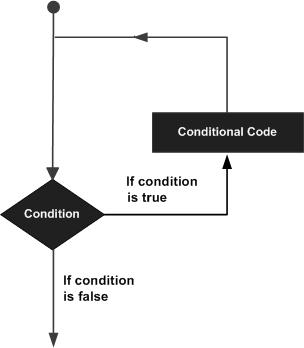The Java programming language provides the following types of loops to handle loop requirements: Click the following link to find out more.

ID Loop and description
1 while loop Repeats a statement or group of statements while certain conditions are true. Test the condition before running the loop body.
2 for Loop Executes a series of statements multiple times to shorten the code that manages loop variables.
3 do ...while loop Similar to the while statement, but tests the condition at the end of the loop body.

## 0209. Java-Conditional branching

There is one or more conditions that are evaluated or tested by the program, a statement that is executed if the condition is determined to be true, and optionally another statement that is executed if the condition is determined. False is. The following is a common form of typical decision-making structure found in most programming languages.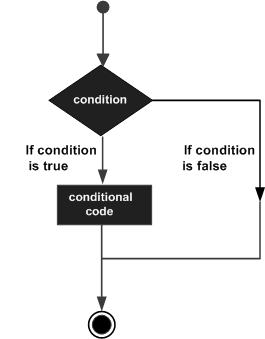The Java programming language provides the following types of decision statements: Click the following link to find out more.

ID Statement and description
1 if statement An if statement consists of a Boolean expression followed by one or more statements.
2 if ...else statement;The if statement can continue with options and is executed when the else statement Boolean expression is false.
3 Nested if statement;You can use one if or else if statement within another if or else if statement.
4 switch statement;Switch statements allow variables to be tested for equality against a list of values.

?? :operator

``````Exp1 ? Exp2 : Exp3;
``````